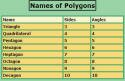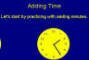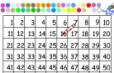Grade 3 Math Review and Practice

 Geometry Name that Polygon& Identify Polygons Area of a RectangleLines of Symmetry Examples of Symmetry in Nature Rounding  & Estimation Rounding to the Nearest 10 Rounding to the Nearest 100 Rounding to the Nearest 1000 Estimating by Rounding Size Estimation Fractions Identify Fractions on a Circle Find Hurkle (Reading Grids) What's the Point Time Elapsed Time Time 30 minute intervals Time 15 minute intervals Time 5 minutes intervals Elapsed TimeTime Quiz Math Mastery Challenge That Quiz Table Trees Fractions less than 1 whole on a number line. Numbers & Patterns Interactive 100's ChartVisualizing Fractions Comparing Fractions with Different Denominators, same numerator Texas Grade 3 Math Test 2003 (self-checking!) Find Grampy (less than 1 whole) Fraction Game Tool Who Wants to be a Mathionarie? Word Problems Hoops